Chemical equilibrium 2013 VCE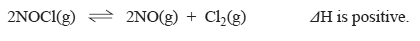1) a)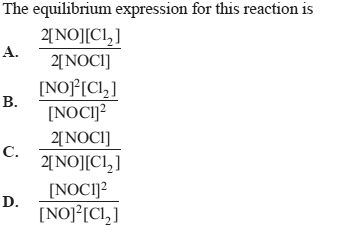Solution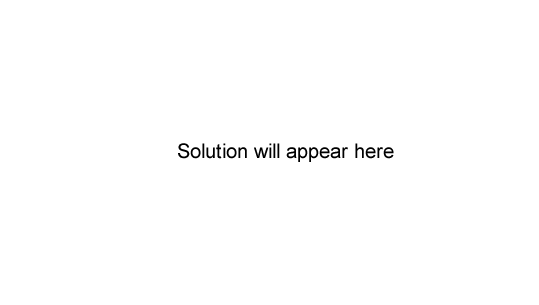b) A concentration–time graph for this system is shown below.
concentration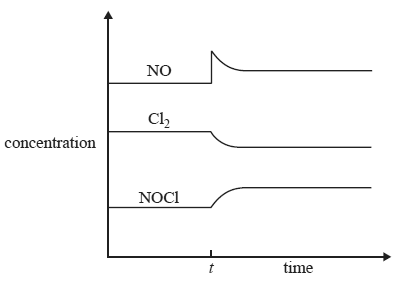What event occurred at time t to cause the change in equilibrium concentrations?
A. The pressure was decreased at a constant temperature.
B. The temperature was increased at a constant volume.
C. A catalyst was added at a constant temperature and volume.
D. Additional NO gas was added at a constant volume and temperature

Solution

2) The industrial production of hydrogen involves the following two reactions.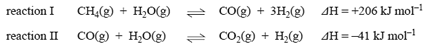a) Write 'increase', 'decrease' or 'no change' in the table below to identify the expected effect of each change to reaction I and reaction II on the equilibrium yield of hydrogen.

 Change to reaction I and reaction II Effect of the change on the hydrogen yield in reaction I Effect of the change on the hydrogen yield in reaction II addition of steam at a constant volume and temperature increase decrease no change increase decrease no change increase in temperature at a constant volume increase decrease no change increase decrease no change addition of a suitable catalyst at a constant volume and temperature increase decrease no change increase decrease no change

Solutionb) Explain the effect of decreasing the volume, at constant temperature, on the hydrogen equilibrium yield in each reaction.

Solution